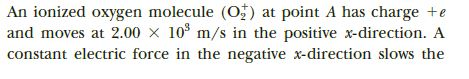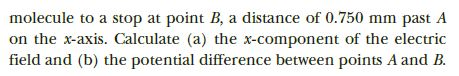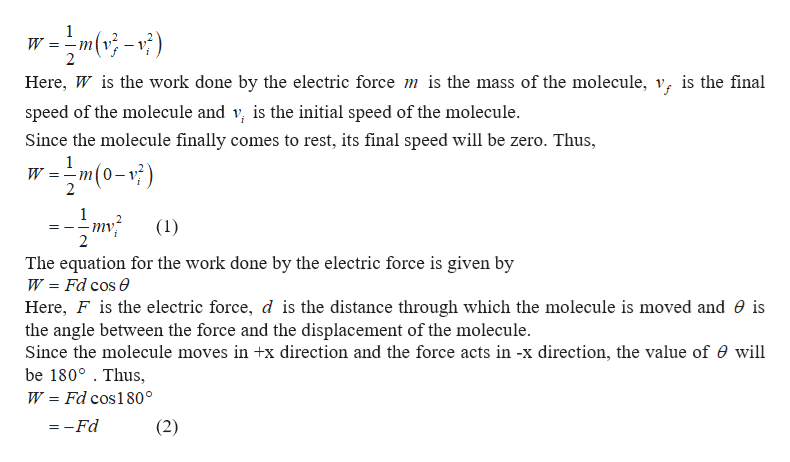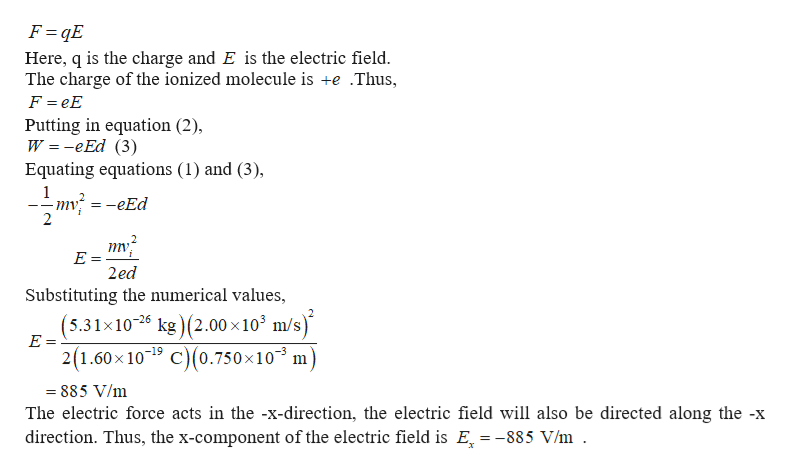# An ionized oxygen molecule (o;) at point A has charge +eand moves at 2.00 x 10 m/s in the positive x-direction. Aconstant electric force in the negative x-direction slows the molecule to a stop at point B, a distance of 0.750 mm past Aon the x-axis. Calculate (a) the x-component of the electricfield and (b) the potential difference between points A and B.

Question
42 viewshelp_outlineImage TranscriptioncloseAn ionized oxygen molecule (o;) at point A has charge +e and moves at 2.00 x 10 m/s in the positive x-direction. A constant electric force in the negative x-direction slows the fullscreenhelp_outlineImage Transcriptionclosemolecule to a stop at point B, a distance of 0.750 mm past A on the x-axis. Calculate (a) the x-component of the electric field and (b) the potential difference between points A and B. fullscreen
check_circle

Step 1

According to the work done by the electric force will be the change in kinetic energy of the ionized oxygen molecule. This can be expressed as,help_outlineImage Transcriptionclosew =mv}-?) Here, W is the work done by the electric force m is the mass of the molecule, v, is the final speed of the molecule and v, is the initial speed of the molecule. Since the molecule finally comes to rest, its final speed will be zero. Thus, -m(0-r;) 2 mv (1) The equation for the work done by the electric force is given by W = Fd cos e Here, F is the electric force, d is the distance through which the molecule is moved and 0 is the angle between the force and the displacement of the molecule. Since the molecule moves in +x direction and the force acts in -x direction, the value of e will be 180° . Thus, W = Fd cos180° = -Fd (2) fullscreen
Step 2

The electric force ...help_outlineImage TranscriptioncloseF = qE Here, q is the charge and E is the electric field. The charge of the ionized molecule is +e .Thus, F = eE Putting in equation (2), W = -eEd (3) Equating equations (1) and (3), -m; = -eEd - -- 2ed Substituting the numerical values, kg )(2.00 ×10° m/s) (5.31x10-6 2(1.60 x 10-19 C)(0.750×10³ m) = 885 V/m The electric force acts in the -x-direction, the electric field will also be directed along the -x direction. Thus, the x-component of the electric field is E, = -885 V/m . fullscreen

### Want to see the full answer?

See Solution

#### Want to see this answer and more?

Solutions are written by subject experts who are available 24/7. Questions are typically answered within 1 hour.*

See Solution
*Response times may vary by subject and question.
Tagged in

### Electric Charges and Fields